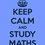# RMO 2014 Delhi Region Q.5

Let $ABC$ be an acute-angeled triangle and let $H$ be its orthocenter. For any point $P$ on the circumcircle of triangle $ABC$, let $Q$ be the point of the intersection of the line $BH$ with the line $AP$ . Show that there is a unique point $X$ on the circumcircle of $ABC$ such that for every point $P\not=A,B$ the circumcircle of $HQP$ pass through $X$.

• You can find rest of the problems here

• You can find the solutions hereNote by Aneesh Kundu
6 years, 5 months ago

This discussion board is a place to discuss our Daily Challenges and the math and science related to those challenges. Explanations are more than just a solution — they should explain the steps and thinking strategies that you used to obtain the solution. Comments should further the discussion of math and science.

When posting on Brilliant:

• Use the emojis to react to an explanation, whether you're congratulating a job well done , or just really confused .
• Ask specific questions about the challenge or the steps in somebody's explanation. Well-posed questions can add a lot to the discussion, but posting "I don't understand!" doesn't help anyone.
• Try to contribute something new to the discussion, whether it is an extension, generalization or other idea related to the challenge.

MarkdownAppears as
*italics* or _italics_ italics
**bold** or __bold__ bold
- bulleted- list
• bulleted
• list
1. numbered2. list
1. numbered
2. list
Note: you must add a full line of space before and after lists for them to show up correctly
paragraph 1paragraph 2

paragraph 1

paragraph 2

[example link](https://brilliant.org)example link
> This is a quote
This is a quote
    # I indented these lines
# 4 spaces, and now they show
# up as a code block.

print "hello world"
# I indented these lines
# 4 spaces, and now they show
# up as a code block.

print "hello world"
MathAppears as
Remember to wrap math in $$ ... $$ or $ ... $ to ensure proper formatting.
2 \times 3 $2 \times 3$
2^{34} $2^{34}$
a_{i-1} $a_{i-1}$
\frac{2}{3} $\frac{2}{3}$
\sqrt{2} $\sqrt{2}$
\sum_{i=1}^3 $\sum_{i=1}^3$
\sin \theta $\sin \theta$
\boxed{123} $\boxed{123}$

Sort by:

I got this! I hope it is right.

- 6 years, 5 months ago

How?

- 6 years, 5 months ago

Take any other point say, $Z$ and let $AZ$ intersect $BH$ at $Y$. And consider circle $HYZ$ meeting circle $ABC$ at $X.$ Now,prove $HQPX$ is a cyclic quad!

- 6 years, 5 months ago

How many are you getting right? (Outta 6, right?)

- 6 years, 5 months ago

I solved 4

- 6 years, 5 months ago

Dunno whether they are right or wrong

- 6 years, 5 months ago

can someone give me the solution to this problem? plzz

- 6 years, 5 months ago

Quite similar to one which came in Rajasthan paper, though more difficult.

- 6 years, 5 months ago